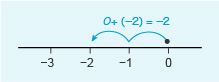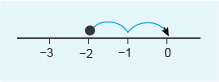Succeed with maths – Part 1

Start this free course now. Just create an account and sign in. Enrol and complete the course for a free statement of participation or digital badge if available.

Free course

# 4 Adding and subtracting negative numbers

You use a similar process to that shown in the previous section, when adding and subtracting negative numbers. Let’s consider a simple case first of 0 + (–2). If you add a number to zero, you end up with the same number, so 0 + 6 = 6. It then follows that the same must be true for our example: 0 + (–2) = –2.

This can be shown on the number line (Figure 15) as follows. Starting at 0 and moving 2 units to the left, the answer is –2. This is the same operation as subtracting 2 from zero as subtraction requires moving to the left in the same way.Figure _unit8.4.1 Figure 15 The sum 0 + (–2) = –2

You now have your first rule to remember when working with negative numbers. Rules in maths are crucial as they give us a way of working with any number – it is always important to use the right rule, at the right time though! Always think carefully if the rule you are using works in the context you are trying to apply it in. The rule is:

• Adding a negative number is the same as subtracting the corresponding positive number.

For example:

30 + (–20) = 30 − 20 = 10 and (–5) + (–10) = (–5) − 10 = –15

How about what happens when you subtract a negative number? This isn’t easy to visualise, so you can try thinking of subtraction in a different way. For example, for the calculation 30 − 20, instead of saying ‘30 take away 20 is …’ you can say, ‘What do I have to add on to 20 to get 30?’. This is finding exactly the same difference but looking at it in a different way.

In the same way, 0 − (–2) can be interpreted as ‘What do I have to add on to (–2) to get 0?’. From the number line in Figure 16, you have to move 2 units to the right to arrive at zero, which is the same as adding on 2. Thus, 0 − (–2) = 2, is in fact the same as 0 + 2.Figure _unit8.4.2 Figure 16 What do I have to add on to –2 to get 0?

• Subtracting a negative number is the same as adding the corresponding positive number.

For example, 30 − (–20) = 30 + 20 = 50 and –30 − (–20) = –30 + 20 = –10.

It’s not easy to see why subtracting a negative number is equivalent to adding a positive number in real life, so think of driving a car along a road, going in a forward direction that you can consider as ‘positive’. If you wanted to go back in the opposite direction, considered as backwards, or ‘negatively’, you have two choices. You could either reverse or turn round. Either is a sort of equivalent to a negative number. But, if the car did both at the same time (that is, turned itself around, and then reversed), then it would continue in the original direction – that is, positively. So, subtraction of a negative number is addition of the positive number.

Now it’s time to practise adding and subtracting negative numbers.

## Activity _unit8.4.1 Activity 5 Adding and subtracting negative numbers

Timing: Allow approximately 10 minutes

Work through these examples without using a calculator. If you need a hint at any point remember you can click on reveal comment.

• a.20 + (–5)

### Discussion

Remember that adding a negative number is the same as subtracting the corresponding positive number.

• a.You are adding a negative number, which is the same as subtracting the corresponding positive number.

20 + (–5) = 20 − 5 = 15

• b.(–20) + (–5)

• b.Again, you are adding a negative number, which is the same as subtracting the corresponding positive number.

(–20) + (–5) = (–20) − 5 = –25

• c.20 – (–5)

### Discussion

Remember that subtracting a negative number is the same as adding the corresponding positive number.

• c.You are subtracting a negative number which is the same as adding the corresponding positive number.

20 − (–5) = 20 + 5 = 25

• d.(–20) − (–5)

• d.Again, you are subtracting a negative number which is the same as adding the corresponding positive number.

(–20) − (–5) = (–20) + 5 = –15

The next section looks at some other methods or rules for addition and subtraction involving negative numbers before moving onto exploring how to use a calculator to help.